## CodeNewbie Community is a community of 10,715 amazing newbiesMiguel Brito

Posted on • Originally published at miguendes.me

# How to Use datetime.timedelta in Python With Examples

In this tutorial, you'll learn how to use `datetime.timedelta` to perforam date arithmetic.

With `timedelta` you can add days, minutes, seconds, hours, weeks and many more to a `datetime.date` or a `datetime.datetime` object.

You'll also learn how to:

• convert a `timedelta` to seconds, minutes, hours, or days
• convert a time delta to years
• how to take the difference between two dates
• how to format a time delta as string

Let's go!

## Adding Seconds, Minutes, Hours, Days, Weeks and Whatnot to a Date

A `timedelta` object denotes a duration, it can also represent the difference between two dates or times.

We can use this object to add or subtract a duration from a `date` and it defines its constructor as `datetime.timedelta(days=0, seconds=0, microseconds=0, milliseconds=0, minutes=0, hours=0, weeks=0)`. As you can see, all arguments are optional and default to 0. It can take `int`s or `float`s, positive or negative.

Even though you can pass weeks, hours, minutes and milliseconds only days, seconds, and microseconds are stored internally.

In this section, we'll see basic arithmetic operations such as adding/subtracting a duration to/from a `date`.

### How to Use `timedelta` to Add Days to a `date` or `datetime` Object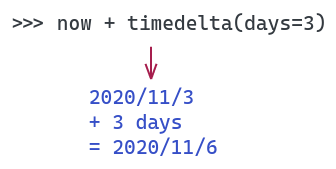Since `timedelta` represents a duration, we can use it to add days to a `datetime`. The number of can be positive or negative, thus allowing us to create a date in the future or in the past. The code snippet below shows an example.

``````>>> import datetime

>>> now = datetime.datetime.now()

>>> now
datetime.datetime(2020, 11, 3, 22, 5, 21, 979147)

>>> from datetime import timedelta

>>> now + timedelta(days=3)
datetime.datetime(2020, 11, 6, 22, 5, 21, 979147)

>>> now + timedelta(days=-3)
datetime.datetime(2020, 10, 31, 22, 5, 21, 979147)
``````

As you can see, adding a positive number of days yields a future date whereas adding a negative number brings the date to the past.

If you want to add days to a `date` object, the process is the same.

``````>>> today = datetime.date.today()

>>> today
datetime.date(2020, 11, 5)

>>> today + timedelta(days=3)
datetime.date(2020, 11, 8)
``````

### How to Use `timedelta` to Add Minutes to a `datetime` Object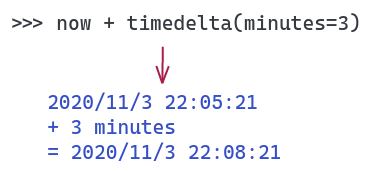Since `timedelta` object sets all arguments to 0 by default, we have the option to set only the ones we need. This allows us to add only minutes, for instance.

``````>>> now
datetime.datetime(2020, 11, 3, 22, 5, 21, 979147)

>>> now + timedelta(minutes=3)
datetime.datetime(2020, 11, 3, 22, 8, 21, 979147)

>>> now + timedelta(minutes=-3)
datetime.datetime(2020, 11, 3, 22, 2, 21, 979147)
``````

### How to Use `timedelta` to Add Weeks, Hours, Seconds, Milliseconds to a `datetime`

Adding weeks, seconds, milliseconds and even microseconds works in a similar fashion.

``````>>> now + timedelta(weeks=3)
datetime.datetime(2020, 11, 24, 22, 5, 21, 979147)

>>> now + timedelta(hours=3)
datetime.datetime(2020, 11, 4, 1, 5, 21, 979147)

>>> now + timedelta(microseconds=3)
datetime.datetime(2020, 11, 3, 22, 5, 21, 979150)

>>> now + timedelta(milliseconds=3)
datetime.datetime(2020, 11, 3, 22, 5, 21, 982147)
``````

### How to Add Years to a `datetime` in Python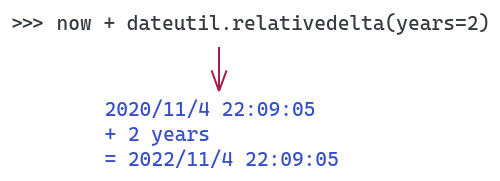It's definitely possible to use `timedelta` to add years to a `datetime`, but some things can go wrong and it's easy to shoot yourself in the foot. For example, you need to take into account leap years yourself. IMHO, the best way to add a certain number of years to a `datetime` is by using the `dateutil` library.

``````>>> import datetime

>>> from dateutil.relativedelta import relativedelta

>>> now = datetime.datetime.now()

>>> now
datetime.datetime(2020, 11, 4, 22, 9, 5, 672091)

>>> now + relativedelta(years=2)
datetime.datetime(2022, 11, 4, 22, 9, 5, 672091)
``````

### How to Add Months to a `datetime` in Python

The same problem arises when we need to add months to a `datetime` using `timedelta`. Adding `months` are not supported by default and requires manual calculation. You can use days, but you’d need to know how many days that month has and so. In a nutshell, it’s too error prone. Again, the best you can do is to use `dateutil.relativedelta`.

``````>>> from dateutil.relativedelta import relativedelta
>>> now
datetime.datetime(2020, 11, 4, 22, 9, 5, 672091)

>>> now + relativedelta(years=2)
datetime.datetime(2022, 11, 4, 22, 9, 5, 672091)

>>> now + relativedelta(months=12)
datetime.datetime(2021, 11, 4, 22, 9, 5, 672091)

>>> now + relativedelta(months=24)
datetime.datetime(2022, 11, 4, 22, 9, 5, 672091)
``````

## How to convert a `timedelta` to seconds, minutes, hours, or days

A `timedelta`object allows adding a delta to a `datetime` but sometimes is useful to convert it into a single time unit, such as seconds, or minutes.

In this section, we'll explore how to do that.

### How to convert a `timedelta` to seconds

A `timedelta` has only one method called `timedelta.total_seconds()`. This method returns the total number of seconds the duration has. If we want to convert a `timedelta` object to seconds, we can just call it.

``````>>> import datetime

>>> delta = datetime.timedelta(days=1, seconds=34)

# a day has 24h, each hour has 60min of 60s = 24*60*60 = 86400
# 86400s + 34s = 86434s
>>> delta.total_seconds()
86434.0
``````

What is the difference between `total_seconds()` and `timedelta.seconds`?

`total_seconds()`—as its name implies—corresponds to total seconds within the whole duration. On the flip side, `timedelta.seconds` is an internal property that represents the number of seconds within a day.

To be more precise, `timedelta.seconds` stores the seconds if it is less than a day, that is, from 0 to 86399. Otherwise, if the number of seconds is greater than 86399, `timedelta` converts this number to days, or weeks as you'll see in the next example.

``````>>> import datetime

>>> delta = datetime.timedelta(seconds=34)

# Delta is withtin 0 and 86399, so delta.seconds returns that number
>>> delta.seconds
34

>>> delta = datetime.timedelta(days=1, seconds=34)

# 1 days + 34s = 86434s, so it overflows to days
>>> delta.seconds
0
>>> delta.days
1
# total_seconds returns 1 day in seconds + 34s = 86434s
>>> delta.total_seconds()
86434.0
``````

### How to convert a `timedelta` to minutes

To convert a `timedelta` to minutes you need to use a bit of math. Unfortunately, `timedelta` does not provide any way of accessing the number of minutes in a duration. In the end, you need to do the conversion yourself.

There are two different ways of doing this conversion:

• the first one you divide the `total_seconds()` by the number of seconds in a minute, which is 60
• the second approach, you divide the `timedelta` object by `timedelta(minutes=1)`
``````>>> import datetime

>>> delta = datetime.timedelta(hours=3, minutes=13, seconds=34)

# there's NO minutes in a time delta object
>>> delta.minutes
---------------------------------------------------------------------------
AttributeError                            Traceback (most recent call last)
<ipython-input-31-b45e912051b9> in <module>
----> 1 delta.minutes

AttributeError: 'datetime.timedelta' object has no attribute 'minutes'

# we set a variable to represent the number of seconds in a minute
>>> NUM_SECONDS_IN_A_MIN = 60

# we then divide the total seconds by the number of seconds in a minute
# this gives us around 193 minutes
>>> delta.total_seconds() / NUM_SECONDS_IN_A_MIN
193.56666666666666

# alternatively, we use the divide operator
>>> delta / datetime.timedelta(minutes=1)
193.56666666666666
``````

### How to convert a `timedelta` to hours

We can follow the same logic to convert a `timedelta` to hours. Instead of dividing the `total_seconds()` by the number of seconds in a minute, or dividing the`timedelta` object by `timedelta(minutes=1)`, we do it for hour.

``````>>> import datetime

>>> delta = datetime.timedelta(hours=3, minutes=13, seconds=34)

delta.hours
--------------------------------------------------------------------------------
AttributeError                            Traceback (most recent call last)
<ipython-input-3-8c2202cab691> in <module>
---------> 1 delta.hours

AttributeError: 'datetime.timedelta' object has no attribute 'hours'

>>> delta / datetime.timedelta(hours=1)
3.226111111111111
``````

### How to convert a `timedelta` to days

Converting a `timedelta` to days is easier, and less confusing, than seconds. According to the docs, only days, seconds and microseconds are stored internally. To get the number of days in a time delta, just use the `timedelta.days`.

⚠️ WARNING: `timedelta.days` is an internal property that is not listed in the docs, so it's not a good idea to rely on it. A more robust approach is to divide the time delta object by `datetime.timedelta(days=1)`.

``````>>> import datetime

>>> delta = datetime.timedelta(weeks=2, days=3, seconds=34)

# 1 week has 7 days, so 2 weeks has 14 days. 2 weeks + 3 days = 17 days
>>> delta.days
17

# if you want the days including the fraction of seconds, divide it by timedelta(days=1)
>>> delta / datetime.timedelta(days=1)
17.000393518518518
``````

### How to convert a `timedelta` to years

If you've been following this guide since the beginning you might have started to pick up a pattern. However, I have bad news.

In the "what is timedelta?" section, I mentioned that you can create a `timedelta` by passing a combination of days, seconds, microseconds, milliseconds, minutes, hours, and weeks.

By default, `timedelta` doesn't support years. To do that we would need to calculate how many weeks there is in how many years we want to pass. It's definitely possible to use `timedelta` to add years to a `datetime`, but some things can go wrong. For example, you need to take into account leap years yourself.

The idea here is to create a variable that holds the number of seconds in a year. A full year has 365 days, but to account for the leap years, we add 0.25 to it, so 365.25. Each day has 24 hours of 60 min, and each minute has 60s. Multiply everything and you get the number of seconds in a year.

``````>>> import datetime

# 1 year has 52 weeks, so we create a delta of 2 years with 2*52
>>> delta = datetime.timedelta(weeks=2*52, days=3, seconds=34)

>>> delta
datetime.timedelta(days=731, seconds=34)

>>> def timedelta_to_years(delta: datetime.timedelta) -> float:
seconds_in_year = 365.25*24*60*60
return delta.total_seconds() / seconds_in_year

>>> timedelta_to_years(delta)
2.0013700027885517

# round to int, if you don't care about the fraction
>>> int(timedelta_to_years(delta))
2
``````

Another alternative—to me the best one—is to get a delta duration in years is by using the `python-dateutil` library.

``````>>> import datetime

>>> from dateutil.relativedelta import relativedelta

>>> delta = relativedelta(years=2, weeks=3, months=1)

>>> delta
relativedelta(years=+2, months=+1, days=+21)

>>> delta.years
2

>>> delta = relativedelta(years=2, weeks=3, months=15)

>>> delta.years
3
``````

## How to Take the Difference Between Two Dates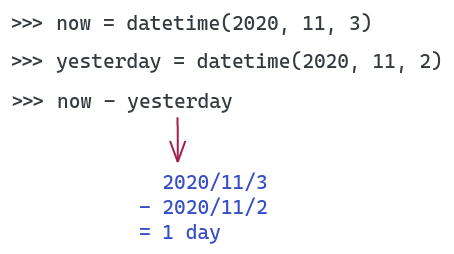As discussed earlier, `timedelta` can also represent the difference between two dates. The following sub-sections illustrate how you can do that.

### How to Calculate the Number of Days Between Two Dates

To obtain the difference between two `datetime` objects in days you can use the `-` operator, for example.

``````>>> now = datetime.datetime.now()

>>> now
datetime.datetime(2020, 11, 3, 22, 36, 21, 674967)

>>> yesterday = datetime.datetime(2020, 11, 2)

>>> now - yesterday
datetime.timedelta(days=1, seconds=81381, microseconds=674967)

>>> now - yesterday
datetime.timedelta(days=1, seconds=81381, microseconds=674967)

>>> (now - yesterday).days
1
``````

### How to Calculate the Number of Minutes Between Two Dates

This one requires more work, and we can achieve it in two different ways. The first one is using `divmod` and the second one is using `timedelta`.

According to the docs , `divmod` takes two (non complex) numbers as arguments and return a pair of numbers consisting of their quotient and remainder when using integer division. In our case, we want to divide the total number of seconds contained in the duration by the number of seconds in one minute, which is 60.

``````>>> now = datetime.datetime.now()

>>> now
datetime.datetime(2020, 11, 3, 22, 57, 12, 300437)

>>> yesterday = datetime.datetime(2020, 11, 2, 22, 57, 12, 300437)

>>> diff = now - yesterday

>>> diff.total_seconds()
86400.0

>>> diff / timedelta(minutes=1)
1440.0

>>> divmod(diff.total_seconds(), 60)
(1440.0, 0.0)

>>> int(diff / timedelta(minutes=1))
1440
``````

## How to Format a `timedelta` as string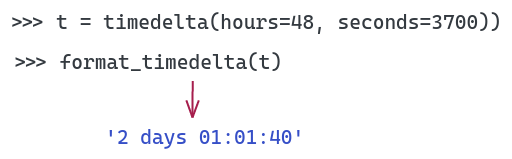Sometimes we want to get a string representation of a `timedelta` object. Even though you can do that by calling `str(timedelta_obj)`, sometimes the result will not be good. The reason is that it can vary depending on the length of the duration the object represents. For example, take a look at what happens when you try to print different `timedelta`s.

``````>>> from datetime import timedelta

>>> timedelta(seconds=123)
datetime.timedelta(seconds=123)

>>> str(timedelta(seconds=123))
'0:02:03'

>>> str(timedelta(seconds=123456))
'1 day, 10:17:36'

>>> str(timedelta(seconds=1234.56))
'0:20:34.560000'
``````

With that in mind, the question is: how can we have a more consistent format?

Sadly, we don’t have many options other than implementing a formatting function ourselves. The good thing is, that’s not so hard.

Suppose we want to print the `timedelta` in this format: `[N days] %H:%M:%S`. One way to do that is using python’s f-strings.

``````def format_timedelta(delta: timedelta) -> str:
"""Formats a timedelta duration to [N days] %H:%M:%S format"""
seconds = int(delta.total_seconds())

secs_in_a_day = 86400
secs_in_a_hour = 3600
secs_in_a_min = 60

days, seconds = divmod(seconds, secs_in_a_day)
hours, seconds = divmod(seconds, secs_in_a_hour)
minutes, seconds = divmod(seconds, secs_in_a_min)

time_fmt = f"{hours:02d}:{minutes:02d}:{seconds:02d}"

if days > 0:
suffix = "s" if days > 1 else ""
return f"{days} day{suffix} {time_fmt}"

return time_fmt
``````
``````>>> format_timedelta(timedelta(hours=23, seconds=3809))
'1 day 00:03:29'

>>> format_timedelta(timedelta(hours=23))
'23:00:00'

>>> format_timedelta(timedelta(hours=25))
'1 day 01:00:00'

>>> format_timedelta(timedelta(hours=48, seconds=3700))
'2 days 01:01:40'
``````

## Conclusion

That’s it for today, folks! I hope you’ve learned something different and useful. Knowing how to perform date calculations such as addition and subtraction is very important. The `timedelta` object is good enough for most situations but if you need more complex operations go for the `dateutil` library.

Other posts you may like:

See you next time!

This post was originally published at https://miguendes.me